Name: ___________________Date:___________________

 Email us to get an instant 20% discount on highly effective K-12 Math & English kwizNET Programs!

### MEAP Preparation - Grade 4 Mathematics1.41 Types of Fractions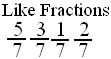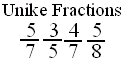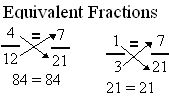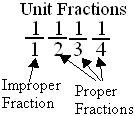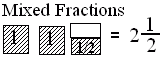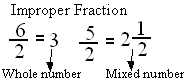Directions: Answer the following questions. Also write at least 5 examples of each type of fractions.
 Q 1: If the numerator is more than the denominator in a fraction, the fraction is called a _________.improper fractionmixed fractionproper fraction Q 2: An improper fraction is sometimes a mixed number or sometimes a whole number.FalseTrue Q 3: Fraction with numerator 1 is called a unit fraction. Are all unit fractions proper?YesNo Q 4: Fractions with same denominators are calledunlike fractionslike fractions Q 5: A fraction less than 1 is called a proper fraction. A fraction equal to one and more than 1 is called _________.improper fractionmixed fractionproper fraction Q 6: A number with a whole number and a proper fraction is aproper numbermixed number Q 7: If the numerator is less than the denominator in a fraction, the fraction is called a _______.improper fractionproper fractionmixed fraction Q 8: Fractions with different denominators are calledunlike fractionslike fractions Question 9: This question is available to subscribers only! Question 10: This question is available to subscribers only!